# C Classes Object Oriented Programming Object Oriented Programming

• Slides: 22C++ Classes & Object Oriented Programming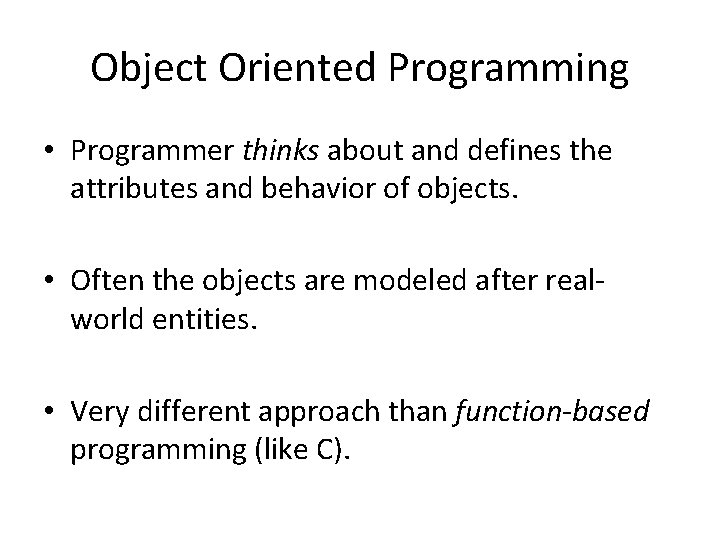Object Oriented Programming • Programmer thinks about and defines the attributes and behavior of objects. • Often the objects are modeled after realworld entities. • Very different approach than function-based programming (like C).Object Oriented Programming • Object-oriented programming (OOP) – Encapsulates data (attributes) and functions (behavior) into packages called classes. • So, Classes are user-defined (programmerdefined) types. – Data (data members) – Functions (member functions or methods) • In other words, they are structures + functions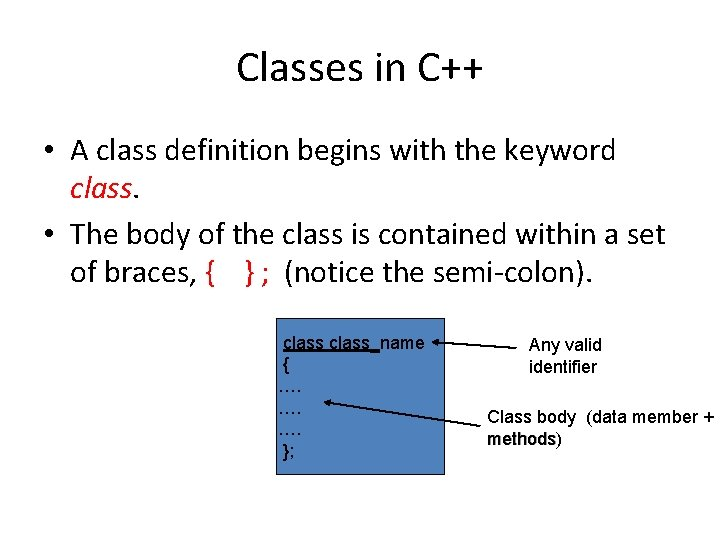Classes in C++ • A class definition begins with the keyword class. • The body of the class is contained within a set of braces, { } ; (notice the semi-colon). class_name { …. …. …. }; Any valid identifier Class body (data member + methods) methods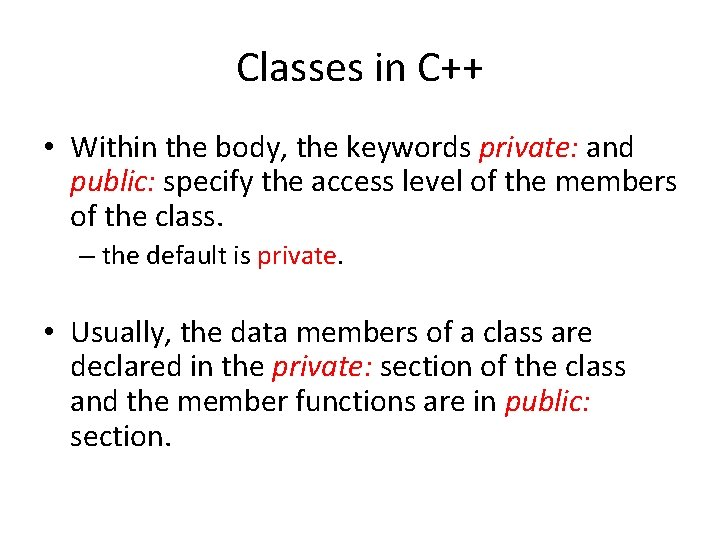Classes in C++ • Within the body, the keywords private: and public: specify the access level of the members of the class. – the default is private. • Usually, the data members of a class are declared in the private: section of the class and the member functions are in public: section.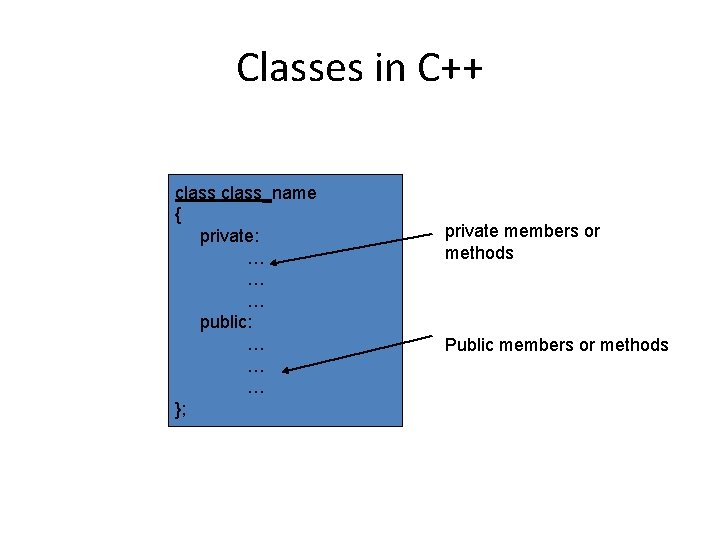Classes in C++ class_name { private: … … … public: … … … }; private members or methods Public members or methodsClasses in C++ • Member access specifiers – public: • can be accessed outside the class directly. – The public stuff is the interface. – private: • Accessible only to member functions of class • Private members and methods are for internal use only.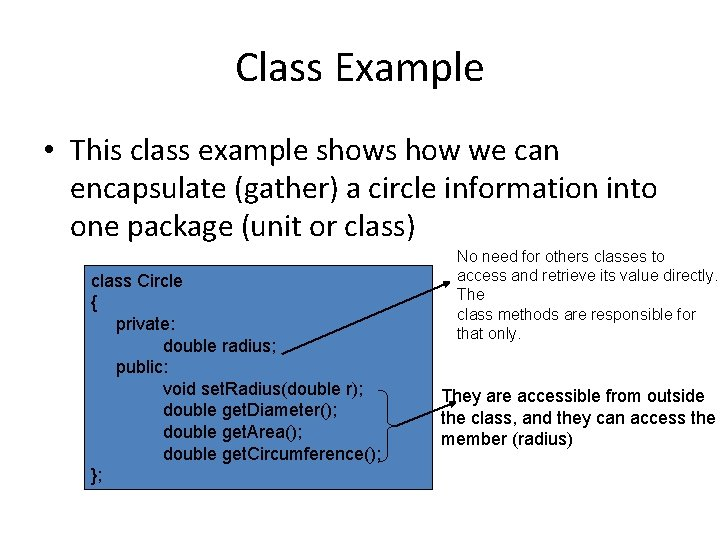Class Example • This class example shows how we can encapsulate (gather) a circle information into one package (unit or class) class Circle { private: double radius; public: void set. Radius(double r); double get. Diameter(); double get. Area(); double get. Circumference(); }; No need for others classes to access and retrieve its value directly. The class methods are responsible for that only. They are accessible from outside the class, and they can access the member (radius)Creating an object of a Class • Declaring a variable of a class type creates an object. You can have many variables of the same type (class). – Instantiation • Once an object of a certain class is instantiated, a new memory location is created for it to store its data members and code • You can instantiate many objects from a class type. – Ex) Circle c; Circle *c;Special Member Functions • Constructor: – Public function member – called when a new object is created (instantiated). – Initialize data members. – Same name as class – No return type – Several constructors • Function overloadingSpecial Member Functions class Circle { private: double radius; public: Circle(); Circle(int r); void set. Radius(double r); double get. Diameter(); double get. Area(); double get. Circumference(); }; Constructor with no argument Constructor with one argument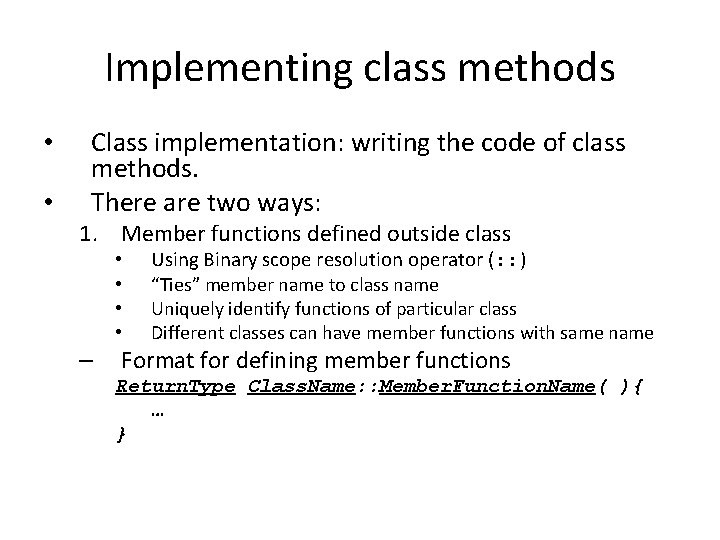Implementing class methods • • Class implementation: writing the code of class methods. There are two ways: 1. Member functions defined outside class • • – Using Binary scope resolution operator (: : ) “Ties” member name to class name Uniquely identify functions of particular class Different classes can have member functions with same name Format for defining member functions Return. Type Class. Name: : Member. Function. Name( ){ … }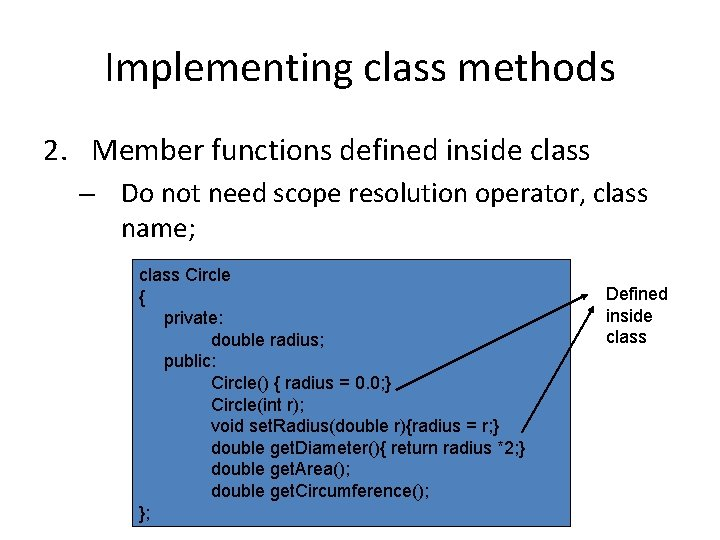Implementing class methods 2. Member functions defined inside class – Do not need scope resolution operator, class name; class Circle { private: double radius; public: Circle() { radius = 0. 0; } Circle(int r); void set. Radius(double r){radius = r; } double get. Diameter(){ return radius *2; } double get. Area(); double get. Circumference(); }; Defined inside class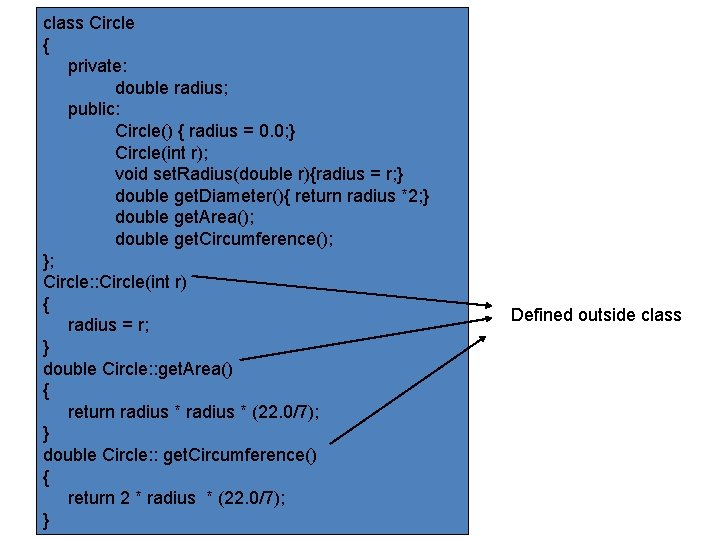class Circle { private: double radius; public: Circle() { radius = 0. 0; } Circle(int r); void set. Radius(double r){radius = r; } double get. Diameter(){ return radius *2; } double get. Area(); double get. Circumference(); }; Circle: : Circle(int r) { radius = r; } double Circle: : get. Area() { return radius * (22. 0/7); } double Circle: : get. Circumference() { return 2 * radius * (22. 0/7); } Defined outside classAccessing Class Members • Operators to access class members – Identical to those for structs – Dot member selection operator (. ) • Object • Reference to object – Arrow member selection operator (->) • Pointers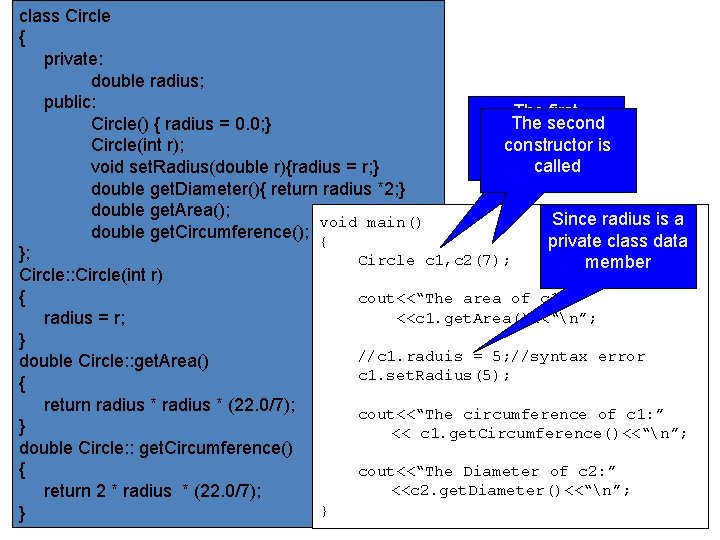class Circle { private: double radius; public: The first The second Circle() { radius = 0. 0; } constructor is Circle(int r); called void set. Radius(double r){radius = r; } double get. Diameter(){ return radius *2; } double get. Area(); Since radius is a void main() double get. Circumference(); { private class data }; Circle c 1, c 2(7); member Circle: : Circle(int r) { cout<<“The area of c 1: ” <<c 1. get. Area()<<“n”; radius = r; } //c 1. raduis = 5; //syntax error double Circle: : get. Area() c 1. set. Radius(5); { return radius * (22. 0/7); cout<<“The circumference of c 1: ” } << c 1. get. Circumference()<<“n”; double Circle: : get. Circumference() { cout<<“The Diameter of c 2: ” <<c 2. get. Diameter()<<“n”; return 2 * radius * (22. 0/7); } }class Circle { private: double radius; public: Circle() { radius = 0. 0; } Circle(int r); void set. Radius(double r){radius = r; } double get. Diameter(){ return radius *2; } double get. Area(); double get. Circumference(); }; void main() Circle: : Circle(int r) { { Circle c(7); radius = r; Circle *cp 1 = &c; } Circle *cp 2 = new Circle(7); double Circle: : get. Area() cout<<“The are of cp 2: ” { <<cp 2 ->get. Area(); return radius * (22. 0/7); } double Circle: : get. Circumference() } { return 2 * radius * (22. 0/7); }Destructors • Destructors – Special member function – Same name as class • Preceded with tilde (~) – – No arguments No return value Cannot be overloaded Before system reclaims object’s memory • Reuse memory for new objects • Mainly used to de-allocate dynamic memory locations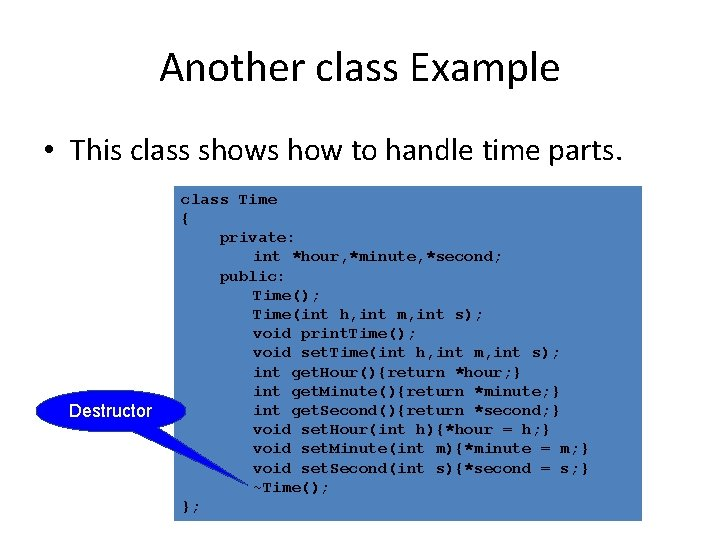Another class Example • This class shows how to handle time parts. Destructor class Time { private: int *hour, *minute, *second; public: Time(); Time(int h, int m, int s); void print. Time(); void set. Time(int h, int m, int s); int get. Hour(){return *hour; } int get. Minute(){return *minute; } int get. Second(){return *second; } void set. Hour(int h){*hour = h; } void set. Minute(int m){*minute = m; } void set. Second(int s){*second = s; } ~Time(); };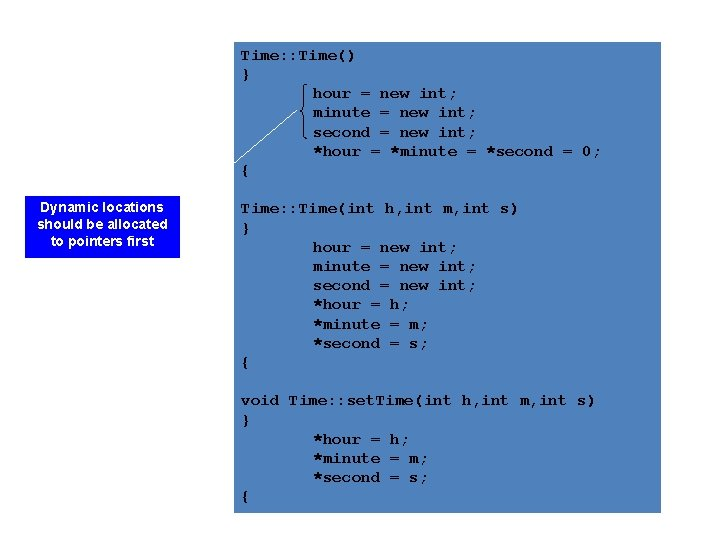Time: : Time() } hour = new int; minute = new int; second = new int; *hour = *minute = *second = 0; { Dynamic locations should be allocated to pointers first Time: : Time(int h, int m, int s) } hour = new int; minute = new int; second = new int; *hour = h; *minute = m; *second = s; { void Time: : set. Time(int h, int m, int s) } *hour = h; *minute = m; *second = s; {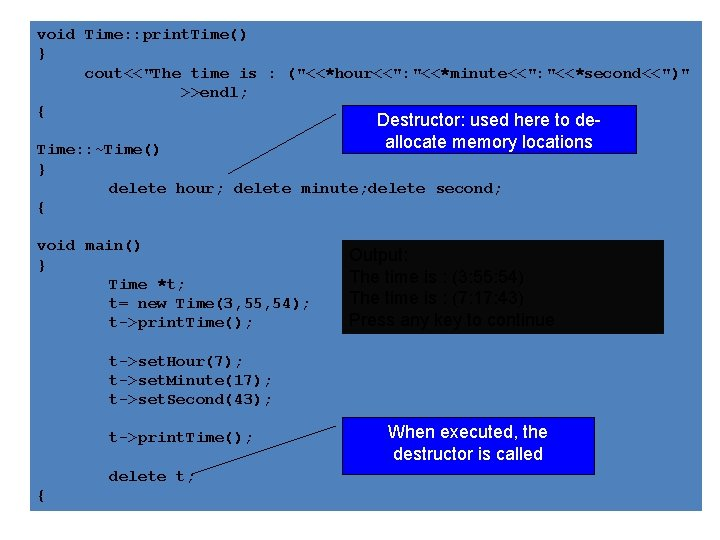void Time: : print. Time() } cout<<"The time is : ("<<*hour<<": "<<*minute<<": "<<*second<<")" >>endl; { Destructor: used here to deallocate memory locations Time: : ~Time() } delete hour; delete minute; delete second; { void main() } Time *t; t= new Time(3, 55, 54); t->print. Time(); Output: The time is : (3: 55: 54) The time is : (7: 17: 43) Press any key to continue t->set. Hour(7); t->set. Minute(17); t->set. Second(43); t->print. Time(); delete t; { When executed, the destructor is calledReasons for OOP 1. Simplify programming 2. Interfaces • Information hiding: – Implementation details hidden within classes themselves 3. Software reuse • Class objects included as members of other classes